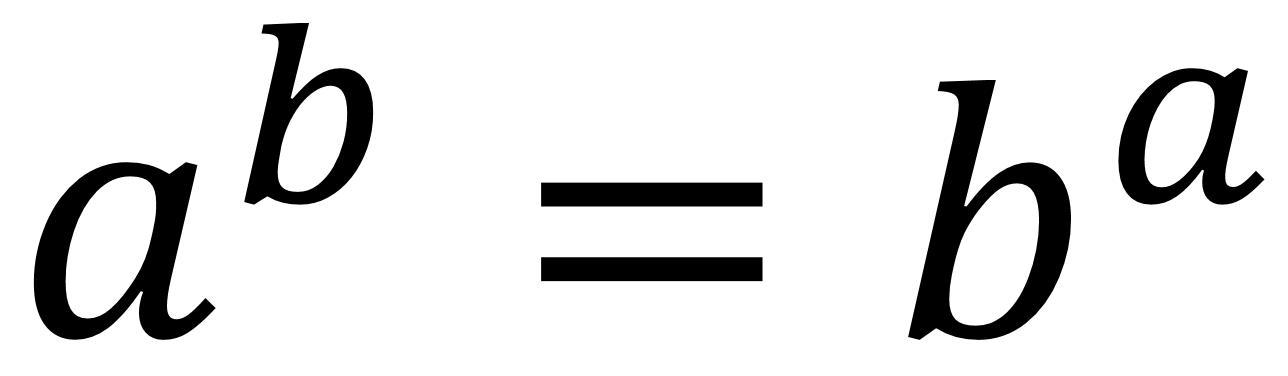Home > Grade 6 > Expressions & Equations > Equivalent Expressions with Powers

# Equivalent Expressions with Powers

Directions: Find values for a and b that will make the expressions equivalent, assuming that a does not equal b.### Hint

How does each base’s factors affect the equivalence?

### Answer

There are two possible answers. One is when a and b are 2 and 4, giving 2^4 = 4^2. The other was found by Shashi and is when a and b are -2 and -4, giving (-2)^(-4) = (-4)^(-2).

Source: Owen Kaplinsky

## Decimal Division

Directions: Using the digits 0 through 9, without repeating any digits, find the quotient closest …

### 4 comments

1.2.Oh! I didn’t think of that!

3.I think have more answer like ;a=1,b=1 this is one answer:a=-1,b=-1

4.Nice one! I’ll add it to the answers.